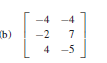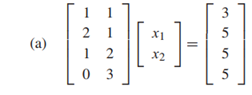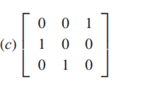﻿ 17074229 4.3-4.4 - 计算数学达人 - 专，学者，数值代数，微分方程数值解

### 17074229 4.3-4.4

4.3 Exercises

2. Apply classical Gram–Schmidt orthogonalization to find the full QR factorization of the

following matrices:Solution:

Set y1=A1=[-4;-2;4],Then r11=||y1||2=sqrt(-(-4^2)+(-2)^2+(4^2))=6.and the first unit vector is q1=y1/||y1||2=[-2/3;-1/3;2/3].to find the second unit vector,set y2=A2-q1*q1’A2=[-4;7;-5]- [-2/3;-1/3;2/3]*(5/3)=[-6;6;-3],and q2= y2/||y2||2=[-2/3;2/3;-1/3],Since r12=q1’*A2=-3,and r22=||y2||2=9

The result written in matrix form is A=[-4 -4;-2 7;4 -5]= [-2/3 -2/3;-1/3 2/3;2/3 -1/3]* [6 3;0 9]=QR

4. Apply modified Gram–Schmidt orthogonalization to find the full QR factorization of the

matrices in Exercise 2.

Solution:

In Exercise 2, we found the orthogonal unit vectors q1=[-2/3;-1/3;2/3] and q2=[-2/3;2/3;-1/3] ,Adding a third vector A3=[1;0;0] leads to y3 = A3 − q1q’A3 − q2q2’A3=9[1;2;2],

And q3=y3/||y3||2=[1;2;2],

Putting the parts together, we obtain the full QR factorization A=[-4 -4;-2 7;4 -5]= [-2/3 -2/3 1;-1/3 2/3 2;2/3 -1/3 2]* [6 3;0 9;0 0]=QR

6. Apply Householder reflectors to find the full QR factorization of the matrices in Exercise 2.

Solution

We need to find a Householder reflector that moves the first column x=[-4;-2;4] to the vector w= [||x||2,0,0]=[6;0;0]. Set v = w − x =[10;2;-4],

H1=I-2P=[1 0 0;0 1 0;0 0 1]-2/120*[100 20 -40;20 4 -8;-40 -8 16]=[-2/3 -1/3 2/3;-1/3 14/15 2/15;2/3 2/15 11/15]

4.3 Computer Problems

1. Write a Matlab program that implements classical Gram–Schmidt to find the reduced QR factorization. Check your work by comparing factorizations of the matrices in Exercise 1 with the Matlab qr(A,0) command or equivalent. The factorization is unique up to signs of the entries of Q and R.

Solution

function [q,r]=QR(A)

[n,m]=size(A);

for j=1:m

y=A(1:n,j);

for i=1:(j-1)

r(i,j)=(q(1:n,i))'*A(1:n,j);

y=y-r(i,j)*q(1:n,i);

end

r(j,j)=norm(y);

q(1:n,j)=y/r(j,j);

end

5. Use the Matlab QR factorization to find the least squares solutions and 2-norm error of the following inconsistent systems:Solution

function [q,r]=QR(A)

[n,m]=size(A);

for j=1:m

y=A(1:n,j);

for i=1:(j-1)

r(i,j)=(q(1:n,i))'*A(1:n,j);

y=y-r(i,j)*q(1:n,i);

end

r(j,j)=norm(y);

q(1:n,j)=y/r(j,j);

end

>>  A=[1 1;2 1;1 2;0 3];b=[3;5;5;5];[q,r]=QR(A)

q =

0.4082    0.0506

0.8165   -0.2025

0.4082    0.3545

0    0.9115

r =

2.4495    2.0412

0    3.2914

>> m=q'*b

m =

7.3485

5.4688

>> x=m*r'

4.4 Exercises

1. Solve Ax = b for the following A and b = [1,0,0] T , using GMRES with x0 = [0,0,0] T . Report all approximations xk up to and including the correct solution.Solution:

X1=[0.0332;0.8505;0.9668],

x2=[0.0672;0.8479;0.9696]

3.let A=[1 0 a13;0 1 a23;0 0 1] Prove that for any x0 and b, GMRES converges to the exact solution after two steps.

Solution:

4.4 Computer Problems

1. Let A be the n × n matrix with n = 1000 and entries A(i, i) = i,A(i, i + 1) = A(i + 1, i) = 1/2,A(i, i + 2) = A(i + 2, i) = 1/2 for all i that fitwithin the matrix. (a) Print the nonzero structure spy(A). (b) Let xe be the vector of n ones. Set b = Axe, and apply the Conjugate Gradient Method, without preconditioner, with the Jacobi preconditioner, and with the Gauss–Seidel preconditioner. Compare errors of the three runs in a plot versus step number.

Solution:

2. Let n = 1000. Start with the n × n matrix A from Computer Problem 1, and add the nonzero entries A(i,2i) = A(2i, i) = 1/2 for 1 ≤ i ≤ n/2. Carry out steps (a) and (b) as in that problem.

Solution: